# DISC Model

This routine implements the DISC correlation function, which is not included by itself as an option in PlantPredict; however, it is used by both the DIRINT decomposition and Hay transposition models.

## Algorithm

1.) Compute the clear-sky normal transmittance for all solar zenith angles.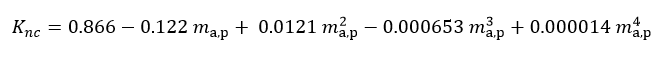2.) Compute coefficients a, b and c for the beam transmittance equation.

1. If Kt ≤ 0.60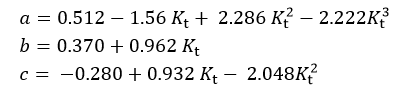1. If Kt > 0.60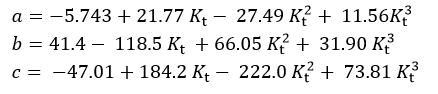3.) Compute the direct normal transmittance.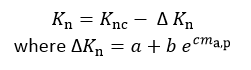4.) Compute the DIRINT direct normal irradiance.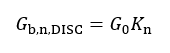5.) Set Gb,n,DISC to 0 if θZ > 87°, GT < 1, or Gb,n,DISC < 0

## Reference

Maxwell, E. L., A Quasi-Physical Model for Converting Hourly Global Horizontal to Direct Normal Insolation.

Technical Report No. SERI/TR-215-3087, Golden, CO: Solar Energy Research Institute, 1987.